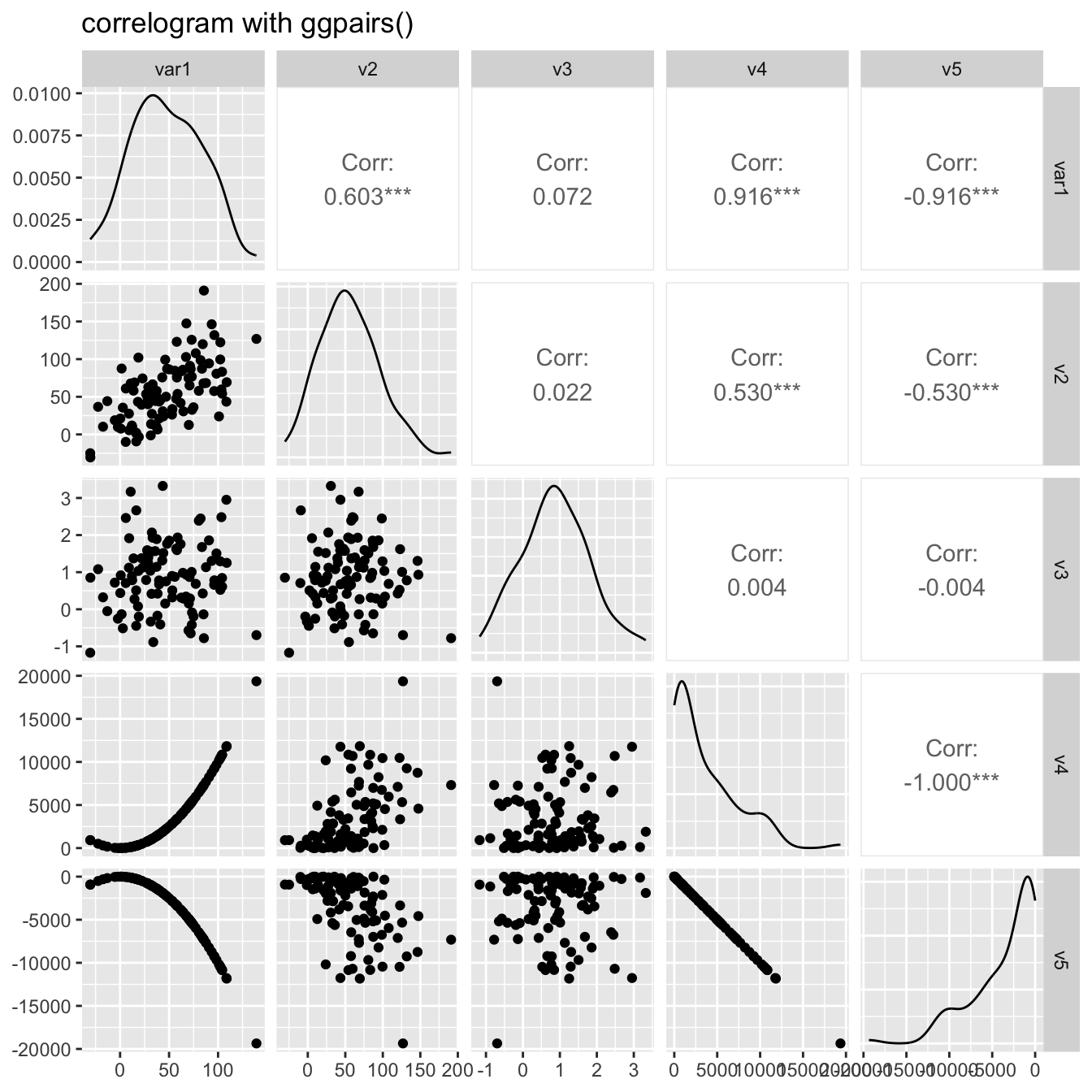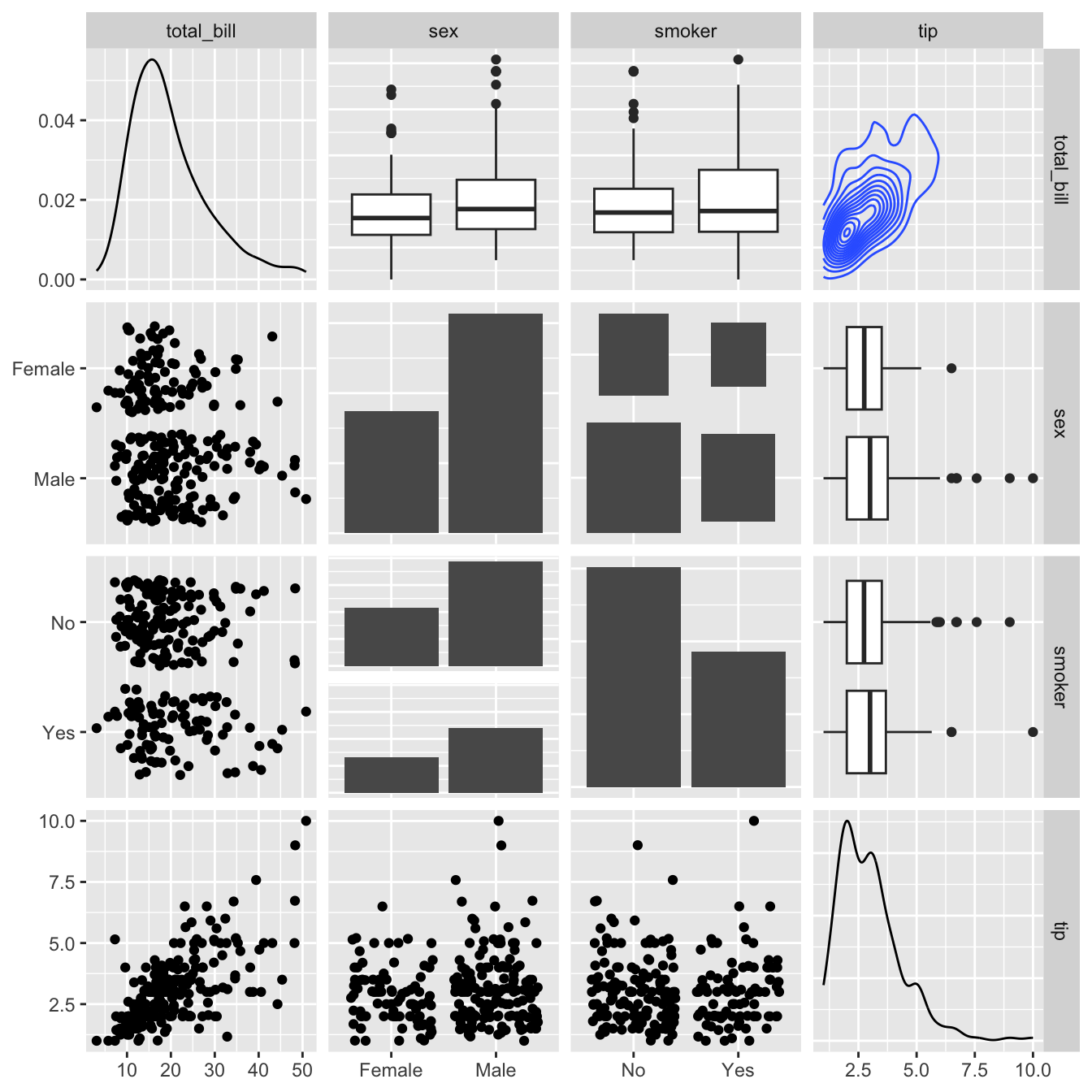# Correlation matrix with ggally

This post explains how to build a correlogram with the `ggally` R package. It provides several reproducible examples with explanation and `R` code.

# Scatterplot matrix with `ggpairs()`

The `ggpairs()` function of the `GGally` package allows to build a great scatterplot matrix.

Scatterplots of each pair of numeric variable are drawn on the left part of the figure. Pearson correlation is displayed on the right. Variable distribution is available on the diagonal.``````# Quick display of two cabapilities of GGally, to assess the distribution and correlation of variables
library(GGally)

# Create data
data <- data.frame( var1 = 1:100 + rnorm(100,sd=20), v2 = 1:100 + rnorm(100,sd=27), v3 = rep(1, 100) + rnorm(100, sd = 1))
data\$v4 = data\$var1 ** 2
data\$v5 = -(data\$var1 ** 2)

# Check correlations (as scatterplots), distribution and print corrleation coefficient
ggpairs(data, title="correlogram with ggpairs()") ``````

# Visualize correlation with `ggcorr()`

The `ggcorr()` function allows to visualize the correlation of each pair of variable as a square. Note that the `method` argument allows to pick the correlation type you desire.``````# Quick display of two cabapilities of GGally, to assess the distribution and correlation of variables
library(GGally)

# Create data
data <- data.frame( var1 = 1:100 + rnorm(100,sd=20), v2 = 1:100 + rnorm(100,sd=27), v3 = rep(1, 100) + rnorm(100, sd = 1))
data\$v4 = data\$var1 ** 2
data\$v5 = -(data\$var1 ** 2)

# Check correlation between variables
#cor(data)

# Nice visualization of correlations
ggcorr(data, method = c("everything", "pearson")) ``````

# Split by group

It is possible to use ggplot2 aesthetics on the chart, for instance to color each category.``````# Quick display of two cabapilities of GGally, to assess the distribution and correlation of variables
library(GGally)

# From the help page:
data(flea)
ggpairs(flea, columns = 2:4, ggplot2::aes(colour=species)) ``````

# Change plot types

Change the type of plot used on each part of the correlogram. This is done with the `upper` and `lower` argument.``````# Quick display of two cabapilities of GGally, to assess the distribution and correlation of variables
library(GGally)

# From the help page:
data(tips, package = "reshape")
ggpairs(
tips[, c(1, 3, 4, 2)],
upper = list(continuous = "density", combo = "box_no_facet"),
lower = list(continuous = "points", combo = "dot_no_facet")
)``````

Related chart types

## Contact

This document is a work by Yan Holtz. Any feedback is highly encouraged. You can fill an issue on Github, drop me a message on Twitter, or send an email pasting yan.holtz.data with gmail.com.